Search IntMath
Close

450+ Math Lessons written by Math Professors and Teachers

5 Million+ Students Helped Each Year

1200+ Articles Written by Math Educators and Enthusiasts

Simplifying and Teaching Math for Over 23 Years

# How to find the equation of a logarithm function from its graph?

By Murray Bourne, 29 May 2019

First, let's revise some of the "typical" shapes a logarithm curve can take. If you're not sure about the background, see the chapter Exponential and Logarithmic Functions.

A logarithm graph of the form y = log10(x) has the following shape: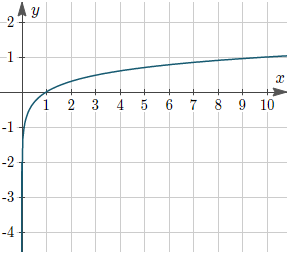Figure 1: The graph of y = log10(x)

Notice the graph passes through the point (1, 0) (since
log(1) = 0, no matter what base we are using) and the point (10, 1), since log10(10) = 1.## The graph of log x using different bases

The following graph shows the cases when the base is:

• 2 (the top-most curve), passing through:
• (4, 2) since log24 = 2, that is, 22 = 4 and
• (8, 3) since log28 = 3, that is 23 = 8;
• e ≈ 2.71828 (the magenta curve);
• 3, passing though (9, 2) since 32 = 9
• 4, 5, 6, 7, 8, 9; and finally
• base 10 (dark green).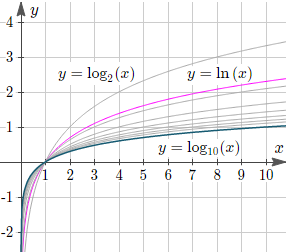Figure 2: The graph of y = logb(x) for various values of b.

## Finding the base from the graph

We can find the base of the logarithm as long as we know one point on the graph. Here, we assume the curve hasn't been shifted in any way from the "standard" logarithm curve, which always passes through (1, 0).

Example: A logarithmic graph, y = logb(x), passes through the point (12, 2.5), as shown. What is the base, b?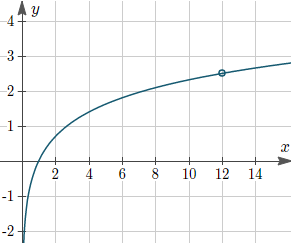Example 1: Find the base

Answer: We substitute in our known values y = 2.5 when x = 12.

2.5 = logb(12)

To find the base, we just need to apply the basic logarithm identity:

If y = logb(x), then

by = x

Applying this, we have:

b2.5 = 12

This gives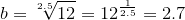So the base of the given logarithm equation is 2.7.

Let's now see some "non-standard" ways the logarithm graph can appear.

## Multiplying the log term

The general form for this curve is:

y = d log10(x)

If we multiply the log term, we elongate (or compress) the graph in the vertical direction. In this next graph, we see from the top-most curve:

d = 5, 4, 3, 2;

y = log10(x) (dark green);

y = 0.5 log10(x)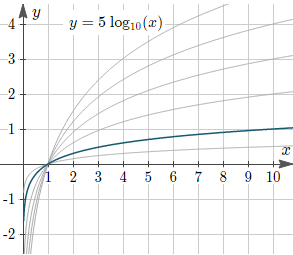Figure 3: Graph of y = d log10(x) for various values of d

While this may look similar to Figure 2 above, it is quite a different situation.

## Reflecting the log graph in the y-axis

Here is the graph of y = log10(−x).

The minus sign before the "x" has the effect of reflecting the curve in the y-axis. We get a vertical mirror image of the curve we saw in Figure 1.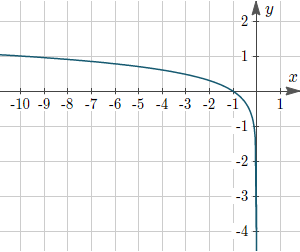Figure 4: Graph of y = log10(−x)

## Reflecting the log graph in the x-axis

Here is the graph of y = log10(x).

The minus sign before the "log(x)" has the effect of reflecting the curve in the x-axis. We get a mirror image horizontally.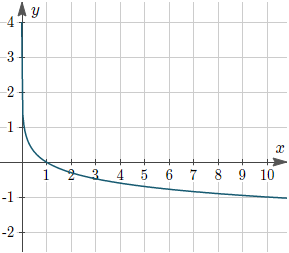Figure 5: Graph of y = log10(x)

## Reflecting the log graph in both the x-axis and y-axis

Here is the graph of y = log10(x).

We get a mirror image across both the vertical and horizontal axes.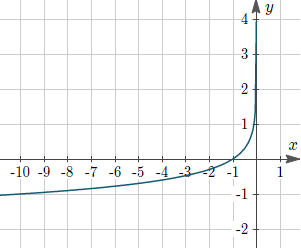Figure 6: Graph of y = log10(x)

NOTE: Compare Figure 6 to the graph we saw in Graphs of Logarithmic and Exponential Functions, where we learned that the exponential curve is the reflection of the logarithmic function in the line y = x. This is not the same situation as Figure 1 compared to Figure 6.

Next, we'll see what happens when we come across logarithm graphs that do not pass neatly though (1, 0) or (−1, 0) as in the cases we've just seen.

## Shifting the logarithm function up or down

We introduce a new formula,

y = c + log(x)

The c-value (a constant) will move the graph up if c is positive and down if c is negative.

For example, here is the graph of y = 2 + log10(x). Notice it passes through (1, 2). It is the curve in Figure 1 shifted up by 2 units.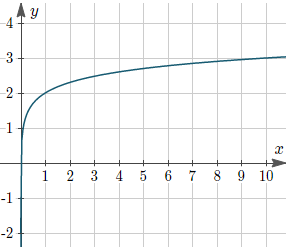Example 2: Graph of y = 2 + log10(x).

Similarly, this is the graph of y = −2 + log10(x). It is the result of shifting the curve in Figure 1 down by 2 units, and it passes through (1, −2).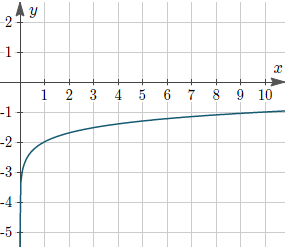Example 3: Graph of y = −2 + log10(x).

## Shifting the logarithm function left or right

We introduce another new formula, where we've replaced x with (x + a):

y = log(x + a)

The "a" will have the effect of shifting the logarithm curve right if a is negative, and to the left if a is positive.

For example, the graph of y = log10(x − 4) looks like: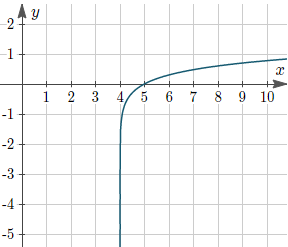Example 4: Graph of y = log10(x − 4)

The curve in Figure 1 has been shifted to the right by 4 units.

Also, the graph of y = log10(x + 5) gives us the curve in Figure 1 moved left by 5 units: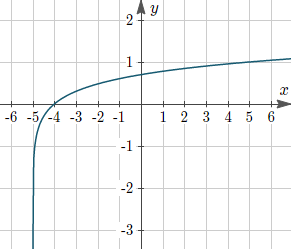Example 5: Graph of y = log10(x + 5)## Summary example

Let's pull what we've learned so far into an example.

Question: Find the equation of the logarithm function (base 10) for the following curve.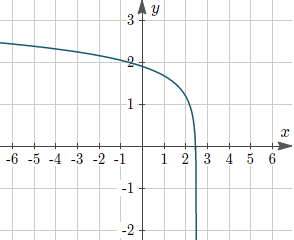Example 6: Find the logarithmic function

Answer: We observe the shape of this curve to be closest to Figure 4, which was y = log10(−x).

We'll assume the general equation is:

y = c + log10(−x + a).

We also observe the (almost) vertical portion of the graph is at x = 2.5, so we replace −x with (x 2.5) and conclude a = 2.5. (Try a few values for x to see why a ends up being positive.)

Here's an interim graph, where I've moved the curve 2.5 units left so I can easily see how high or low the graph is: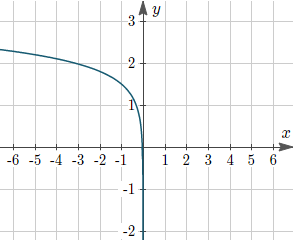Example 6a: Interim graph, moved 2.5 units left

We know the graph needs to pass through (−1, 0), and we observe we are 1.5 units too high. So we conclude c = 1.5.

So the required equation is

y = 1.5 + log10(−x + 2.5).

## Determining the equation of a logarithmic function from data

There are many computer packages (SPSS, Excel, etc) that can determine a "best fit" curve for a given set of data. But for the sake of explaining how to determine an unknown logarithmic function from its data plot, let's take a look at this example (one which is close to my heart).

The experiment: A group of people are asked to learn a list of random words. They are tested immediately, then again after some time has elapsed, and then repeatedly over longer time spans. As expected, the number of words they remember correctly diminishes over time.

The average data is plotted over time as follows, where the horizontal axis is time, and the vertical axis is the proportion of the words they got right. (This is the famous Ebbinghaus Forgetting Curve.)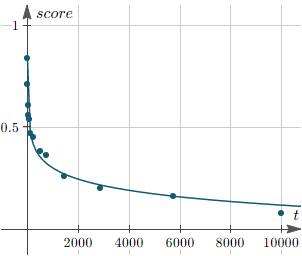Example 7: Ebbinghaus Forgetting data

The question: You can see a "best fit" curve has been drawn through the data points. What is the equation of that curve?

The answer: We observe it is most like Figure 5 , which had the formula y = log10(x).

We assume our logarithmic function will have the form:

y = c − d log10(x).

We now aim to find the values of c and d.

We know from the section on Graphs on Logarithmic and Semi-Logarithmic Axes that we can turn a logarithmic (or exponential) curve into a linear curve by taking the logarithm of one of the variables.

To do so, we take the original data set (the columns "time" and "proportion") and find the logarithm of the independent variable, time. (Normally we would use natural log for such analysis, but I'm sticking with base 10 to be consistent with the earlier examples.)

time
(t)
log10(t) proportion
1 0 0.84
5 0.69897 0.71
15 1.17609 0.61
30 1.47712 0.56
60 1.77815 0.54
120 2.07918 0.47
240 2.38021 0.45
480 2.68124 0.38
720 2.85733 0.36
1440 3.15836 0.26
2880 3.45939 0.2
5760 3.76042 0.16
10080 4.00346 0.08

Now we can easily deduce the linear equation for this curve (I'm taking the first data point and the second last, since the last one is clearly not that close to the smooth curve.)

I use the point-slope formula for a line: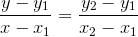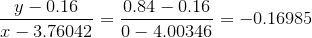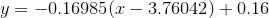y = 0.79872 0.16985 x

Next, we simply replace the "x" with log10(x) and achieve the required equation:

y = 0.79872 0.16985 log10(x)

NOTE: The Ebbinghaus Forgetting curve is usually modeled using an exponential curve, but this data quite nicely fits a logarithmic curve for the given values. It's not a good model if we extrapolate it into the future, since this logarithmic curve doesn't flatten out (like the appropriate exponential one would, and it eventually goes off into negative territory.)

[Data credit: Penn State]

## Conclusion

Hopefully, whatever logarithmic graph you are trying to find the equation for will be covered by one or more of the cases above.### 3 Comments on “How to find the equation of a logarithm function from its graph?”

1. Alan Cooper says:

The problem with using a logarithm (of time) to approximate the forgetting data is that it eventually predicts a negative proportion of correct answers.

2. Murray says:

@Alan Yes, I mentioned we usually use an exponential curve for forgetting data. I added a bit more of a caution just now.

3. Joe says:

Regarding the last example: Is x1 = 3.76042 or 4.00346?

### Comment Preview

HTML: You can use simple tags like <b>, <a href="...">, etc.

To enter math, you can can either:

1. Use simple calculator-like input in the following format (surround your math in backticks, or qq on tablet or phone):
a^2 = sqrt(b^2 + c^2)
(See more on ASCIIMath syntax); or
2. Use simple LaTeX in the following format. Surround your math with $$ and $$.
$$\int g dx = \sqrt{\frac{a}{b}}$$
(This is standard simple LaTeX.)

NOTE: You can mix both types of math entry in your comment.